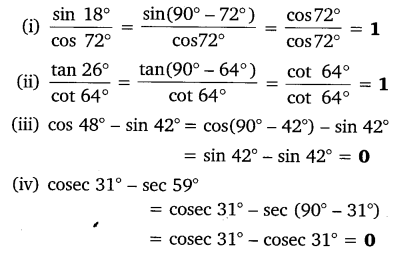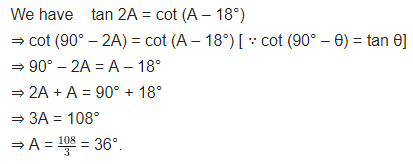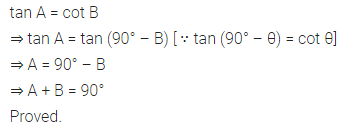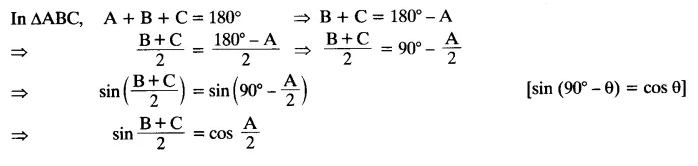ncert textbook

# NCERT Solutions For Class 10 Maths Chapter 8 Introduction to Trigonometry Ex 8.3

Get Free NCERT Solutions for Class 10 Maths Chapter 8 Ex 8.3 Introduction to Trigonometry Class 10 Maths NCERT Solutions are extremely helpful while doing homework. Exercise 8.3 Class 10 Maths NCERT Solutions were prepared by Experienced ncert-books.in Teachers. Detailed answers of all the questions in Chapter 8 Maths Class 10 Coordinate Geometry Exercise 8.3 Provided in NCERT Textbook.NCERT Solutions For Class 10 Maths Chapter 8 Introduction to Trigonometry Ex 8.3

NCERT Solutions for Class 10 Maths Chapter 8 Introduction to Trigonometry Ex 8.3 are part of NCERT Solutions for Class 10 Maths. Here we have given NCERT Solutions for Class 10 Maths Chapter 8 Introduction to Trigonometry Ex 8.3.

Question 1.
Evaluate:Solution:Question 2.
Show that:
(i) tan 48° tan 23° tan 42° tan 67° = 1
(ii) cos 38° cos 52° – sin 38° sin 52° = 0
Solution:Question 3.
If tan 2A = cot (A – 18°), where 2A is an acute angle, find the value of A.
Solution:Question 4.
If tan A = cot B, prove that A + B = 90°.
Solution:Question 5.
If sec 4A = cosec (A – 20°), where 4A is an acute angle, find the value of A.
Solution:Question 6.
If A, B and C are interior angles of a triangle ABC, then show that: sin ($\frac { B+C }{ 2 }$) = cos$\frac { A }{ 2 }$
Solution:Question 7.
Express sin 61° + cos 75° in terms of trigonometric ratios of angles between 0° and 45°.
Solution: